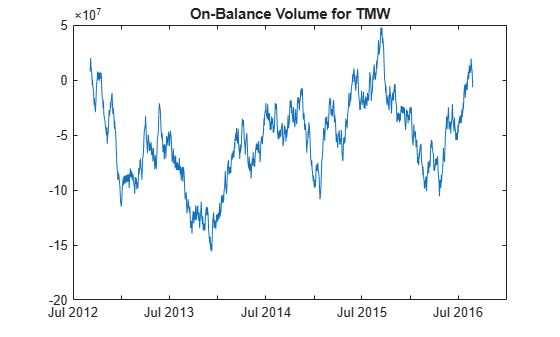# onbalvol

On-Balance Volume (OBV)

## Syntax

``volume = onbalvol(Data)``

## Description

example

````volume = onbalvol(Data)` calculates the On-Balance Volume from the series of closing stock prices and trade volume.```

## Examples

collapse all

Load the file `SimulatedStock.mat`, which provides a timetable (`TMW`) for financial data for TMW stock.

```load SimulatedStock.mat volume = onbalvol(TMW); plot(volume.Time,volume.OnBalanceVolume) title('On-Balance Volume for TMW')```## Input Arguments

collapse all

Data for closing prices and trade volume, specified as a matrix, table, or timetable. For matrix input, `Data` is an `M`-by-`2` matrix of closing prices and trade volume stored in the first and second columns. Timetables and tables with `M` rows must contain variables named `'Close'` and `'Volume'` (case insensitive).

Data Types: `double` | `table` | `timetable`

## Output Arguments

collapse all

On-Balance Volume, returned with the same number of rows (`M`) and the same type (matrix, table, or timetable) as the input `Data`.

 Achelis, S. B. Technical Analysis from A to Z. Second Edition. McGraw-Hill, 1995, pp. 207–209.